Cortix 2019 07Aug2019

# Droplet Swirl Example¶

• This is part of the Cortix-on-Jupyter-Notebook examples.
• You must be in a Jupyter Notebook server to run this notebook.
• Select each of the cells below and run them sequentially (use the run button, >| on the tool bar or use the Cell option on the menu bar).
• Alternatively, on the menu bar run all cells: Cell -> Run All.

$\newcommand{\Amtrx}{\boldsymbol{\mathsf{A}}} \newcommand{\Bmtrx}{\boldsymbol{\mathsf{B}}} \newcommand{\Smtrx}{\boldsymbol{\mathsf{S}}} \newcommand{\xvec}{\boldsymbol{\mathsf{x}}} \newcommand{\vvar}{\boldsymbol{v}} \newcommand{\fvar}{\boldsymbol{f}} \newcommand{\Power}{\mathcal{P}} \newcommand{\bm}{{\boldsymbol{#1}}}$

## Introduction¶

This Cortix use-case simulates the motion of a swarm of droplets in a vortex stream. It consists of two modules, namely, a Droplet module used to model the droplet dynamics, and a Vortex module used to model the effects of the surrounding air on the falling droplets. The Droplet module is instantiated as many times as there are droplets in the simulation while a single Vortex module is connected to all Droplet instances. The communication between modules entails a two-way data exchange between the Vortex module and the Droplet modules, where Droplet sends
its position to Vortex and Vortex returns the air velocity to Droplet at the given position.

### Droplet motion model¶

The equation of motion of a spherical droplet can be written as: \begin{equation*} m_\text{d}\,d_t\bm{v} = \bm{f}_\text{d} + \bm{f}_\text{b} , \end{equation*} where \begin{equation*} \bm{f}_\text{d} = c_\text{d} A \, \rho_\text{f}\, \frac{||\bm{v} - \bm{v}_\text{f}||}{2}\,(\bm{v} - \bm{v}_\text{f}) , \end{equation*} is the form drag force on the droplet, \begin{equation*} \bm{f}_\text{b} = (m_\text{d} - m_\text{f})\,g \hat{z} , \end{equation*} is the buoyancy force on the droplet, \begin{equation*} c_\text{d}(Re) = \begin{cases} \frac{24}{Re} & Re < 0.1\\ \Bigl(\sqrt{\frac{24}{Re}} + 0.5407\Bigr)^2 & 0.1 \leq Re < 6000 \\ 0.44 & Re \geq 6000 \end{cases} \end{equation*} is the drag coefficient as a function of Reynold's number, $Re=\frac{\rho_\text{f}\,||\bm{v}||\,d}{\mu_\text{f}}$. The mass of the droplet and its displaced fluid mass are denoted $m_d$ and $m_f$, respectively. Droplet diameter, $d$, dynamic viscosity, $\mu_\text{f}$, and mass density, $\rho_\text{f}$, of the surrounding air are provided.

### Vortex motion model¶

Here we simply use an imposed vortex circulation in analytical form given by its tangential component of velocity \begin{equation*} v_\theta(r,z,t) = \Bigl(1 - e^{\frac{-r^2}{8\,r_c^2}}\Bigr) \frac{\Gamma}{2\pi\, \max(r,r_c)} f(z) \, \bigl|\cos(\mu\,t)\bigr|, \end{equation*} and its vertical component \begin{equation*} v_z(z,t) = v_h \, f(z) \, \bigl|\cos(\mu\,t)\bigr|, \end{equation*} where \begin{equation*} f(z) = e^{\frac{-(h - z)}{\ell}} \end{equation*} is a vertical relaxation factor, $r_c$ is the vortex core radius, $\Gamma = \frac{2\pi R}{v_\theta |_{r = R}}$ is the vortex circulation, $R$ is the vortex outer radius, $h$ is the height of the vortex, and $\ell$ is the relaxation length of $v_z$.

## Write the run context¶

In :
# Import various packages; must have the Cortix repository installed

import scipy.constants as const
import matplotlib.pyplot as plt

# Leave this block here for Azure
try:
import cortix
except ImportError:
print('Installing the "cortix" package...')
print('')
!pip install cortix
import cortix

from cortix.src.cortix_main import Cortix
from cortix.src.network import Network

# Import the example modules
from cortix.examples.droplet_swirl.vortex import Vortex
from cortix.examples.droplet_swirl.droplet import Droplet

In :
# Create a Cortix object with Python multiprocessing

swirl = Cortix(use_mpi=False,splash=True)

swirl.network = Network()
swirl_net = swirl.network

# Set parameters in SI units

n_droplets = 15
end_time = 3*const.minute
time_step = 0.2

 2019-10-23 14:44:56,843 - cortix - INFO - Created Cortix object
_____________________________________________________________________________
L A U N C H I N G
_____________________________________________________________________________
...                                        s       .     (TAAG Fraktur)
xH88"~ .x8X                                 :8      @88>
:8888   .f"8888Hf        u.      .u    .      .88      %8P      uL   ..
:8888>  X8L  ^""   ...ue888b   .d88B :@8c    :888ooo    .     [email protected]  @88R
X8888  X888h        888R Y888r ="8888f8888r -*8888888  [email protected]  ""Y888k/"*P
88888  !88888.      888R I888>   4888>"88"    8888    888E    Y888L
88888   %88888      888R I888>   4888> "      8888      888E      8888
88888 > 8888>     888R I888>   4888>        8888      888E      888N
8888L %  ?888   ! u8888cJ888   .d888L .+    .8888Lu=   888E   .u./"888&
8888  -*""   /   "*888*P"    ^"8888*"     ^%888*     888&  d888" Y888*"
"888.      :"      "Y"          "Y"         "Y"      R888"  "Y   Y"
""***~"                                           ""
https://cortix.org
_____________________________________________________________________________

In :
# Create the application network

# Vortex module (single)
vortex = Vortex()
swirl_net.module(vortex)
vortex.show_time = (True,1*const.minute)
vortex.end_time = end_time
vortex.time_step = time_step

for i in range(n_droplets):

# Droplet modules (multiple)
droplet = Droplet()
swirl_net.module(droplet)
droplet.end_time = end_time
droplet.time_step = time_step
droplet.bounce = False    # allow droplets to bounce off the ground
droplet.slip = False          # allow droplets to slip on the ground (otherwise will stick)
droplet.save = True         # Save the simulation data for post-processing

swirl_net.connect( [droplet, 'external-flow'], [vortex, vortex.get_port('fluid-flow:{}'.format(i))], 'bidirectional')


## Verify the network connectivity¶

In :
# View the Cortix network created

swirl_net.draw()

Out:

## Run network simulation¶

In :
# Run the simulation!

swirl.run()

 2019-10-23 14:44:57,071 - cortix - INFO - Launching Module <cortix.examples.droplet_swirl.vortex.Vortex object at 0x1516ff3f98>
 2019-10-23 14:44:57,076 - cortix - INFO - Launching Module <cortix.examples.droplet_swirl.droplet.Droplet object at 0x1516ff3f60>
 2019-10-23 14:44:57,079 - cortix - INFO - Vortex::time[min] = 0.0
 2019-10-23 14:44:57,083 - cortix - INFO - Launching Module <cortix.examples.droplet_swirl.droplet.Droplet object at 0x1517016518>
 2019-10-23 14:44:57,093 - cortix - INFO - Launching Module <cortix.examples.droplet_swirl.droplet.Droplet object at 0x1517040f98>
 2019-10-23 14:44:57,101 - cortix - INFO - Launching Module <cortix.examples.droplet_swirl.droplet.Droplet object at 0x1149b93c8>
 2019-10-23 14:44:57,112 - cortix - INFO - Launching Module <cortix.examples.droplet_swirl.droplet.Droplet object at 0x15170509b0>
 2019-10-23 14:44:57,123 - cortix - INFO - Launching Module <cortix.examples.droplet_swirl.droplet.Droplet object at 0x1517050fd0>
 2019-10-23 14:44:57,133 - cortix - INFO - Launching Module <cortix.examples.droplet_swirl.droplet.Droplet object at 0x15170545c0>
 2019-10-23 14:44:57,146 - cortix - INFO - Launching Module <cortix.examples.droplet_swirl.droplet.Droplet object at 0x1517054ba8>
 2019-10-23 14:44:57,157 - cortix - INFO - Launching Module <cortix.examples.droplet_swirl.droplet.Droplet object at 0x1517059160>
 2019-10-23 14:44:57,172 - cortix - INFO - Launching Module <cortix.examples.droplet_swirl.droplet.Droplet object at 0x1517050e80>
 2019-10-23 14:44:57,184 - cortix - INFO - Launching Module <cortix.examples.droplet_swirl.droplet.Droplet object at 0x1517059080>
 2019-10-23 14:44:57,197 - cortix - INFO - Launching Module <cortix.examples.droplet_swirl.droplet.Droplet object at 0x1517059e10>
 2019-10-23 14:44:57,210 - cortix - INFO - Launching Module <cortix.examples.droplet_swirl.droplet.Droplet object at 0x1517064438>
 2019-10-23 14:44:57,222 - cortix - INFO - Launching Module <cortix.examples.droplet_swirl.droplet.Droplet object at 0x1517064a20>
 2019-10-23 14:44:57,236 - cortix - INFO - Launching Module <cortix.examples.droplet_swirl.droplet.Droplet object at 0x1517064e80>
 2019-10-23 14:45:06,060 - cortix - INFO - Vortex::time[min] = 1.0
 2019-10-23 14:45:15,457 - cortix - INFO - Vortex::time[min] = 2.0
 2019-10-23 14:45:25,302 - cortix - WARNING - Network::run(): not all modules reloaded from disk.
 2019-10-23 14:45:25,303 - cortix - INFO - run()::Elapsed wall clock time [s]: 28.46


## Results inspection through Cortix¶

### All droplets¶

In :
'''All droplets' trajectory'''

from mpl_toolkits.mplot3d import Axes3D
import matplotlib.pyplot as plt

position_histories = list()
for m in swirl_net.modules[1:]:
position_histories.append( m.liquid_phase.get_quantity_history('position').value )

fig = plt.figure(1)
ax.set_title('Droplet Trajectories')
ax.set_xlabel('x')
ax.set_ylabel('y')
ax.set_zlabel('z')
for p in position_histories:
x = [u for u in p]
y = [u for u in p]
z = [u for u in p]
ax.plot(x,y,z)
plt.rcParams['figure.figsize'] = [10,10]
plt.show()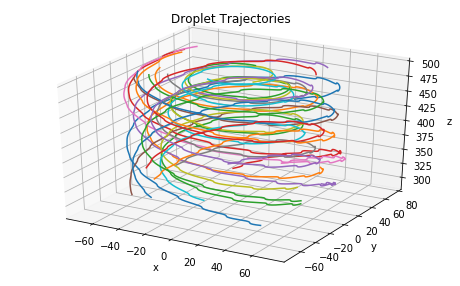Trajectories of all droplets released from random positions at 500-m altitude. Multiprocessing parallel run with number of processes corresponding to all modules: all Droplet modules, 1 Vortex module, and 1 Cortix master process.

In :
'''All droplets' speed'''

fig = plt.figure(2)
plt.xlabel('Time [min]')
plt.ylabel('Speed [m/s]')
plt.title('All Droplets')

for m in swirl_net.modules[1:]:
speed = m.liquid_phase.get_quantity_history('speed').value
plt.plot(list(speed.index/60), speed.tolist())

plt.rcParams['figure.figsize'] = [8,5]
plt.grid()
plt.show()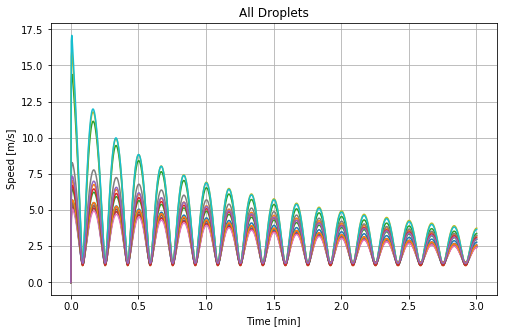In :
'''All droplets' radial position'''

fig = plt.figure(3)
plt.xlabel('Time [min]')
plt.title('All Droplets')

for m in swirl_net.modules[1:]:

plt.rcParams['figure.figsize'] = [8,5]
plt.grid()
plt.show()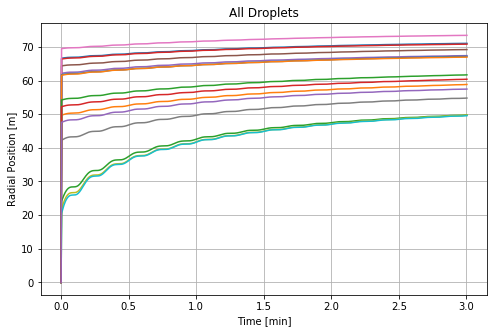### Individual droplet modules¶

In :
'''Droplet 1 Module State'''

droplet_1 = swirl_net.modules
(speed_quant,time_unit) = droplet_1.liquid_phase.get_quantity_history('speed')
print('time unit = ',time_unit)
print('speed unit = ',speed_quant.unit)
speed = speed_quant.value
speed.plot(title='Droplet 1: Speed vs Time')
plt.rcParams['figure.figsize'] = [8,5]
plt.grid()
plt.show()

time unit =  s
speed unit =  m/s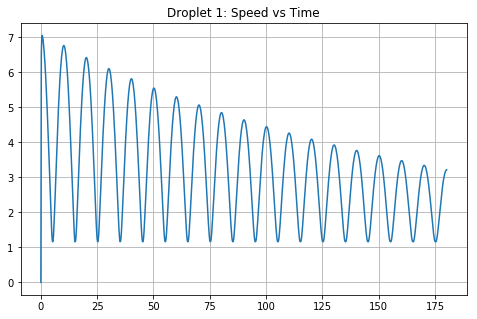In :
(radial_position_quant,time_unit) = droplet_1.liquid_phase.get_quantity_history('radial-position')
print('time unit = ',time_unit)
plt.rcParams['figure.figsize'] = [8,5]
plt.grid()
plt.show()

time unit =  s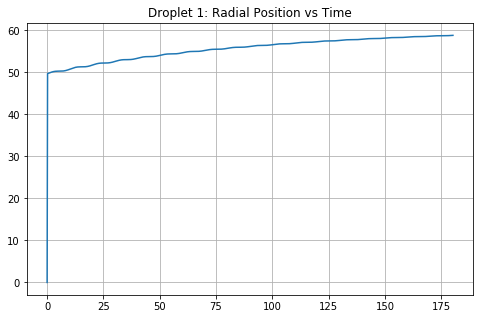# Droplet at Scale¶

Below is a graph of the number of droplets in the system vs the elapsed time of the corresponding simulation. This scaling experiment uses randomly positioned droplets at altitude of 500 m. Therefore the work for each run is not exactly the same since time integration of the trajectories use time adaptivity and some trajectories take more work to complete than others. However the plot below shows the trend of the one-to-all communication bottlenect with increasing number of droplet modules.

Data collected courtesy of the Idaho National Labs HPC center (https://hpc.inl.gov)

In :
import matplotlib.pyplot as plt

# Droplet number vs Simulation runtimes
droplet_run_times = [(250, 127), (500, 168), (1000, 346), (2000, 1660), (3000, 2659.24)]

# Calculate the average runtime per droplet
drops_per_sec = [x/y for (x,y) in droplet_run_times]
avg_drop_per_sec = sum(drops_per_sec) / len(drops_per_sec)
print("Average number of droplets handled per second: %.2f" % avg_drop_per_sec)

plt.plot([x for (x, y) in droplet_run_times], [y for (x, y) in droplet_run_times])
plt.title("Wall-Clock Time vs Number of Droplets")
plt.xlabel("Number of Droplets (MPI processes or Cores)")
plt.ylabel("Wall-Clock Time (s)")
plt.grid()
plt.savefig("droplet_trend.png")
plt.show()

Average number of droplets handled per second: 2.03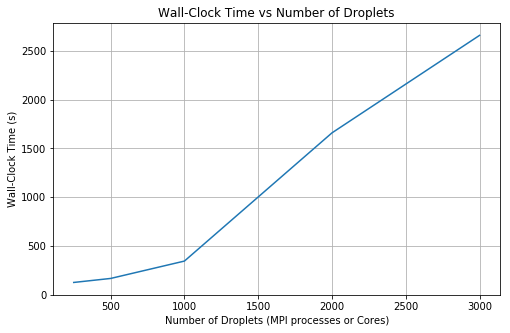In [ ]: